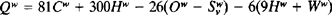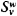# Heat Value

## heat value

[′hēt ‚val·yü]
(physical chemistry)
McGraw-Hill Dictionary of Scientific & Technical Terms, 6E, Copyright © 2003 by The McGraw-Hill Companies, Inc.
The following article is from The Great Soviet Encyclopedia (1979). It might be outdated or ideologically biased.

## Heat Value

(or calorific value, heat of combustion), the quantity of heat liberated upon the complete combustion of a unit weight or unit volume of fuel. It is expressed in, for example, kilojoules (kJ) or kilocalories (kcal) per kg or m3. In Great Britain and the USA, heat values are often measured in Btu/lb; 1 Btu/lb = 2.326 kJ/kg. The heat value is an important indicator of the usefulness of a fuel. Heat values are determined by calorimetry.

If, after combustion, the water originally contained in the fuel and the water formed from the burning of the hydrogen in the fuel are present in liquid form, the quantity of heat liberated is characterized by the high, or gross, heat value Qh. If the water is in the form of vapor, the heat liberated is characterized by the low, or net, heat value Ql. The relation between the high and low heat values is given by the equation

QI = QhK(W + 9H)

Here, W is the amount of water in the fuel, in percent of the total weight; H is the amount of hydrogen in the fuel, in percent of the total weight; and k is a constant equal to 25 kJ/kg, or 6 kcal/kg.

In some countries, such as the USSR and the Federal Republic of Germany, the low heat value is generally used in thermal calculations. In the USA, Great Britain, and France, the high heat value is used.

Heat values may be defined in special ways. For example, Qw is the heat value with respect to the working weight of the fuel, that is, the weight of the fuel in the form in which it is supplied to the user; Qd is the heat value with respect to the dry weight of the fuel; and Qc is the heat value with respect to the combustible weight of the fuel, that is, the weight of the fuel excluding moisture and ash.

In approximate calculations, heat values are found from empirical formulas. For example, the heat values of solid and liquid fuels can be calculated from Mendeleev’s formulawhere Cw, Hw, Ow,, and Ww are the amounts, in percent, of carbon, hydrogen, oxygen, volatile sulfur, and water, respectively, in the total working weight of the fuel.

Comparative calculations make use of a standard fuel having the heat value 29,308 kJ/kg, or 7,000 kcal/kg.

I. N. ROZENGAUZ

References in periodicals archive ?
The ICI 4TM index is the benchmark for Indonesian thermal coal with a low heat value that is widely used in Asia-Pacific for heating and power generation.
It is noted that a gallon of gasoline has a heat value of 0.138 GJ while a gallon of 85: 15 blend has a heat content of 0.130 with a reduction of 5.4% in heat content.
As cited by Ramey, Lumley, Prieto, Porter, and Cath (2015), the higher heat value (HHV) is the value where the water vapors present in the fuel gases are condensed and the lower heat value (LHV) is the value at which these vapors do not condense.
The price will be applicable on gross heat value basis.
11) in beginning combustion phase in the case of Simulation 2 is significantly lower than in diesel (Simulation 1), the contrary situation occurs in the case of Simulation 3, where lowered amount of reaction heat value can be observed, what let conclude about lower thermal loads occuences for this kind of solution.
This creates close to two times as much heat value and can be easily achieved just by stacking up the wood and keeping it covered to protect it from rain, but where the wind can get to it for another year.
* Lower heating value: the heat value of a combustion process excluding latent heat from vaporization of water.
Addressing the India Energy Congress here, Jaiswal stated that the move of the government towards adopting Gross Calorific Value (GCV) based grading and pricing of thermal coals in place of the earlier Useful Heat Value (UHV) based system is a step forward towards this direction.
Recent coal washability test work, performed by the internationally accredited Stewart Laboratory in Ulaanbaatar, indicated that a product with 6500 kcal/kg heat value, less than 10% ash and 0.5% sulphur (all air dried basis) can be produced with high plant yields.
The basic quality parameters of oil shale are lower heat value as mined ([Q.sup.w]) and grain-size range.
When generator's running heat value is at maximum, house-box's of hydrogenerator's cooling capacity does not become enough for that running condition.

Site: Follow: Share:
Open / Close